# Randomizing Sliding Puzzle Tiles

JavaScript

Share:

#### Free JavaScript Book!Write powerful, clean and maintainable JavaScript.

RRP \$11.95

In a previous tutorial, I demonstrated how to create a sliding puzzle game with HTML5 canvas.

To save time I hard-coded the starting tile positions. Game play would be better if the tiles were randomized, but doing so would have led to complications that would require a separate tutorial to explain.

This is that tutorial.

There are a number of ways to randomize the tiles. I’ll look at a few options and discuss their strengths and weaknesses, as well as the problems that arise and how to overcome them.

One simple method is to initialize the puzzle in a solved state, then repeatedly call a function to slide a random piece into the empty space.

``````function initTiles() {
var slideLoc = new Object;
var direction = 0;
for (var i = 0; i < 30; ++i) {
direction = Math.floor(Math.random()*4);
slideLoc.x = emptyLoc.x;
slideLoc.y = emptyLoc.y;
if (direction == 0 && slideLoc.x > 0) {
slideLoc.x = slideLoc.x - 1;
} else if (direction == 1 && slideLoc.y > 0) {
slideLoc.y = slideLoc.y - 1;
} else if (direction == 2 && slideLoc.x < (tileCount - 1)) {
slideLoc.x = slideLoc.x + 1;
} else if (direction == 3 && slideLoc.y < (tileCount - 1)) {
slideLoc.y = slideLoc.y + 1;
}
slideTile(emptyLoc, slideLoc);
}
}``````

In this case we’re sliding 30 tiles, twice the total number of tiles in the 4×4 puzzle, and yet most of the pieces remain in their original locations. To get anything resembling randomness we would need many more iterations.

That’s not an efficient way to randomize the puzzle. Ideally, we’d like to move each piece only once. We could initialize the puzzle to a solved state, then iterate through the tiles, swapping each one with a tile chosen at random.

``````function initTiles() {
for (var i = 0; i < tileCount; ++i) {
for (var j = 0; j < tileCount; ++j) {
var k = Math.floor(Math.random() * tileCount);
var l = Math.floor(Math.random() * tileCount);
swapTiles(i, j, k, l);
}
}
}

function swapTiles(i, j, k, l) {
var temp = new Object();
temp = boardParts[i][j];
boardParts[i][j] = boardParts[k][l];
boardParts[k][l] = temp;
}``````

Not only does this method give us a much more random-looking configuration, it does so in fewer lines of code. This algorithm, however, has two serious flaws. The first problem is subtle. Although swapping each tile with a random location is much more efficient than simply sliding pieces into the empty slot, this still is not a truly random algorithm. Some starting positions will show up much more frequently than others.

In a 2×2 puzzle, some starting configurations will occur 87% more often than others. Add a third row and some configurations appear five times as often as others—and it continues to get worse as more tiles are added. Fortunately, there’s a way to achieve true randomness without adding extra complexity. It’s known as the Fisher-Yates algorithm.

``````function initTiles() {
var i = tileCount * tileCount - 1;
while (i > 0) {
var j = Math.floor(Math.random() * i);
var xi = i % tileCount;
var yi = Math.floor(i / tileCount);
var xj = j % tileCount;
var yj = Math.floor(j / tileCount);
swapTiles(xi, yi, xj, yj);
--i;
}
}``````

The mathematics of the Fisher-Yates are beyond the scope of this tutorial, but it does give every tile an equal chance to appear in any square. Using this algorithm, the puzzle is as random as the `Math.random()` function can get.

But swapping tiles randomly—with the Fisher-Yates algorithm or any other—leads to another problem. Half of all possible tile configurations give us a puzzle that can never be solved. To prevent unleashing an unsolvable puzzle on an innocent user, we need yet another algorithm.

Before I introduce this algorithm, I need to define two terms: inversion and polarity. An inversion is a pair of tiles that are in the reverse order from where they ought to be. The polarity of a puzzle is whether the total number of inversions among all tiles is even or odd. A puzzle with 10 inversions has even polarity; a puzzle with 7 inversions has odd polarity.

The solved puzzle has zero inversions (and even polarity) by definition. If we swapped two neighboring tiles from a solved puzzle, we would have one inversion.

In this game the board is configured as a two-dimensional array, each piece represented by its x/y coordinates.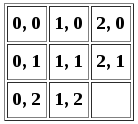But to work with inversions and polarity we’ll think of it as a one-dimensional array. We can convert each tile’s coordinates to a single number n with the formula n = y * w + x, where w is the width. Pictured as a single-dimension array the tiles are numbered like this.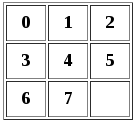Now let’s consider a randomized puzzle. It might look like this.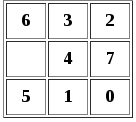There are 19 inversions. Tile 6 is inverted with all six of the tiles numbered 0 through 5; 3 is inverted with 0, 1, and 2; 2 is inverted with 0 and 1; 4 is inverted with 0 and 1; 7 is inverted with 0, 1 and 5; 5 is inverted with 0 and 1; and 1 is inverted with 0.

To get this total, we need a function to count the inversions for each tile.

``````function countInversions(i, j) {
var inversions = 0;
var tileNum = j * tileCount + i;
var lastTile = tileCount * tileCount;
var tileValue = boardParts[i][j].y * tileCount + boardParts[i][j].x;
for (var q = tileNum + 1; q < lastTile; ++q) {
var k = q % tileCount;
var l = Math.floor(q / tileCount);

var compValue = boardParts[k][l].y * tileCount + boardParts[k][l].x;
if (tileValue > compValue && tileValue != (lastTile - 1)) {
++inversions;
}
}
return inversions;
}``````

Now we can iterate through the tiles and keep a running sum of the inversions.

``````function sumInversions() {
var inversions = 0;
for (var j = 0; j < tileCount; ++j) {
for (var i = 0; i < tileCount; ++i) {
inversions += countInversions(i, j);
}
}
return inversions;
}``````

Sliding a tile sideways does not change the number of inversions; the empty square has no number, so swapping it with an adjacent tile will always leave us with the same number of inversions. However, we might change the number of inversions when sliding a tile up or down. For example, if we slide the 6 tile down, we reduce the number of inversions from 19 to 17.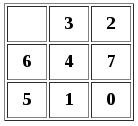The rule is that sliding a tile up or down will change its relationship with w – 1 tiles, where w is the width of the puzzle. So for the 3×3 puzzle, we are changing the tile’s relationship with two other tiles. This may result in a reduction of two inversions, an increase of two inversions, or no change. In the puzzle above, for example, sliding tile 5 up would have left us with 19 inversions, as it would gain an inversion with 4 and lose an inversion with 7.

A puzzle that starts with an even number of inversions will always have an even number of inversions; a puzzle with an odd number of inversions will always have an odd number of inversions. This is true not just for the 3×3 puzzle, but for any puzzle with an odd width. If we’re ever going to reach zero inversions, we must start with an even number.

Since we’ve already calculated the number of inversions, a simple function will tell us whether the puzzle is solvable.

``````function isSolvable() {
return (sumInversions() % 2 == 0)
}``````

The example above is not solvable, since 19 is not even. But suppose the first two tiles were reversed?Now we start with 18 inversions. The 3 and 6 are no longer inverted, but everything else remains the same. We have a solvable puzzle.

This gives us an elegant solution that preserves the puzzle’s true randomness—every unsolvable puzzle is paired with a unique solvable puzzle that differs only in the first two tiles.

``````if (!isSolvable()) {
swapTiles(0, 0, 1, 0);
initEmpty();
}``````

Unfortunately, this won’t work if one of the swapped tiles is the empty square. We’ll need special code to deal with that situation.

``````if (!isSolvable()) {
if (emptyLoc.y == 0 && emptyLoc.x <= 1) {
swapTiles(tileCount - 2, tileCount - 1, tileCount - 1, tileCount - 1);
} else {
swapTiles(0, 0, 1, 0);
}
initEmpty();
}``````

If the empty square is in one of the first two locations, we instead swap the last two tiles. This slightly skews the randomness, but we’re still much closer than any other algorithm can get us.

There’s just one problem remaining. If the width of the puzzle is an even number, sliding a tile up or down reverses the polarity. This is because, as we saw above, the tile changes its relationship with w – 1 tiles.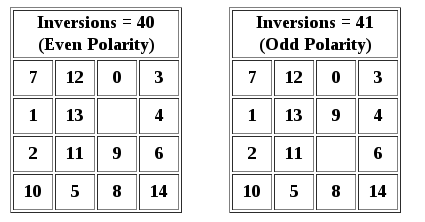In order for the puzzle to be solvable, it must have an even polarity when the empty square is on the bottom row (assuming the empty square is on the bottom row when the puzzle is solved). When the empty square is on the next row up, the puzzle is solvable if the polarity is odd. So for an even-width puzzle, we must sum the inversions plus the distance between the empty row and the bottom row.

``````function isSolvable(width, height, emptyRow) {
if (width % 2 == 1) {
return (sumInversions() % 2 == 0)
} else {
return ((sumInversions() + height - emptyRow) % 2 == 0)
}
}``````

Now we must edit the line that calls this function.

``if (!isSolvable(tileCount, tileCount, emptyLoc.y + 1))``

There are a couple things to note here.

First, because the `emptyLoc` array is zero-based, we need to add one before comparing it with the height.

Second, for a square puzzle we don’t technically need two parameters for height and width; they are the same value, and we’re passing the `tileCount` variable to both. But separating them in the function clarifies which dimension is used in each equation. If we were to make a rectangular puzzle, we’d know where to use width and where to use height.

Randomizing a sliding puzzle turns out to be more work than creating the puzzle in the first place, but it’s worth the effort for the better game play it provides. You can see an example of a randomized puzzle here.### Bruce Alderman

Bruce Alderman is Lead Programmer for Johnson County Library in Kansas and a freelance writer. He has been building web sites since 1997.

#### New books out now!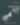Learn how Git works, and how to use it to streamline your workflow!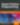Google, Netflix and ILM are Python users. Maybe you should too?